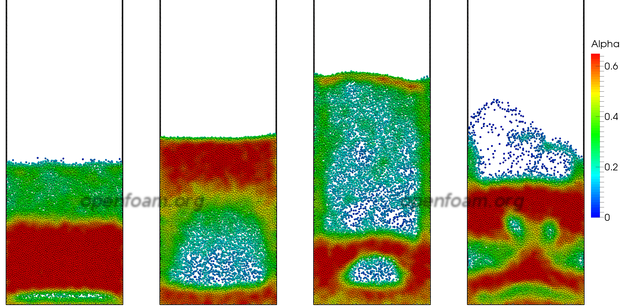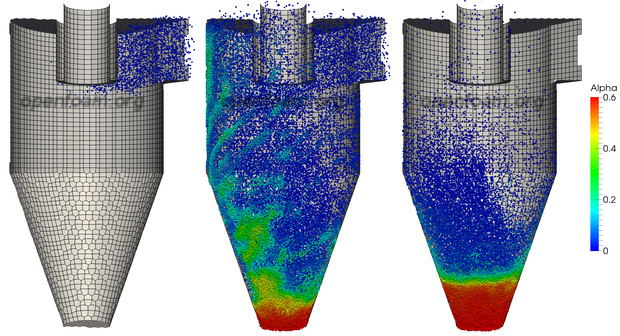Close

# OpenFOAM 2.3.0: Discrete Particle Modelling

## Dense Particle Flows

OpenFOAM includes the DPMFoam solver which includes the effect of the particulate volume fraction on the continuous phase, suitable for dense particle flow simulation. The solver uses existing functionality for particle clouds and their collisions, which directly resolves particle-particle interactions.

#### Source code

• DPMFoam solver
`\$FOAM``_SOLVERS/lagrangian/DPMFoam`

#### Example

• Goldschmidt experimental case
`\$FOAM``_TUTORIALS/lagrangian/DPMFoam/Goldschmidt`

## Particle Drag Modelling

The new version of OpenFOAM includes particle drag models that are suitable for dense particle flows:

• `WenYu`, suitable for particulate volume fractions up to approximately 0.2;
• `ErgunWenYu`, suitable for all particulate volume fractions up to the closed packed condition;
• `PlessisMasliyah`, suitable for all particulate volume fractions up to the closed packed condition.

The images below show results from a DPM simulation of the Goldschmidt fluidised bed. The simulation uses approximately 24,000 spherical particles, shown to scale in the images below, coloured by particulate volume fraction. The simulation uses the `ErgunWenYu` drag model, which gives good prediction of the bed lift.#### Source code

• lagrangianIntermediate library
`\$FOAM``_SRC/lagrangian/intermediate`

#### Example

• Fluidised bed
`\$FOAM``_TUTORIALS/lagrangian/MPPICFoam/Goldschmidt`

## Collision Handling and MP-PIC

This version includes an implementation of the Multiphase Particle-in-Cell (MP-PIC) method for collisional exchange, according to P. J. O’Rourke et al., Chemical Engineering Science 64:1784-1797, 2009. The functionality is implemented into a new MPPIC particle cloud which is used in the new MPPICFoam solver (which is identical to DPMFoam, except it used a colliding particle cloud). Particle-particle interactions are represented by models which utilise mean values calculated on the Eulerian mesh.

There is a choice of methods of computing and interpolating an average of the Lagrangian phase:

• `basic` – simple averaging over cell volumes, suitable for simple cases with no packing;
• `dual` – averaging over the cells and dual volumes, which results in a small gradient basis to improve stability for packed volume fractions.

Packing models are designed which apply the highly non-linear inter-particle stress to the parcels in a stable manner.

• `explicit` – uses the old-time volume fraction to calculate the force, and then limits the resulting impulse to that of a rebound with a coefficient of restitution; this method results in realistic motion of the particle field, but does not guarantee that the cloud will not over-pack;
• `implicit` – calculates the force by solving an implicit equation for the new-time particle volume fraction; the particulate volume fraction is much more effectively kept within the specified threshold, but some excess scattering can occur if there are few particles per cell.

There are a number of models for the inter-particle stress, which depend primarily on the particulate volume fraction: `Harris-Crighton`; `Lun`; and, `exponential`.

Collisional damping models are included to represent the mean loss in kinetic energy which occurs as particles collide. The code includes a `relaxation` model which uses a time-scale to calculate the rate of interaction of a particle’s momentum with that of the mean field. This helps produce physically realistic scattering behaviour, but can have a detrimental affect on the packing modelling.

Collisional isotropy models represent the scattering which occurs as a result of particle collisions. The code includes a `stochastic` model which uses a time-scale to calculate the probability of a particle undergoing a collision, which randomises its velocity. Momentum and energy are explicitly conserved as a second step. This approach also helps to spread the particles uniformly across cells, which improves the quality of computed averages.

The images below show an MPPIC simulation of cyclone, with implicit packing and isotropy modelling. There are approximately 0.9M parcels, each representing 0.5M particles, most of which collect in the packed region. Such a simulation, of almost half a billion particles would be impractical using DEM. Sphere sizes are not representative, and the images plot only 10% of the parcels, coloured by particulate volume fraction.#### Source code

• lagrangianIntermediate library
`\$FOAM``_SRC/lagrangian/intermediate/submodels/MPPIC`
• MPPICFoam solver
`\$FOAM``_SOLVERS/lagrangian/DPMFoam/MPPICFoam`

#### Examples

• Cyclone
`\$FOAM``_TUTORIALS/lagrangian/MPPICFoam/cyclone`

17th February 2014